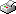Print This Page Annika Tillander -- doctoral thesis -- Classifcation models for high-dimensional data with sparsity patterns Abstract Today's high-throughput data collection devices, e.g. spectrometers and gene chips, create information in abundance. However, this poses serious statistical challenges, as the number of features is usually much larger than the number of observed units. Further, in this high-dimensional setting, only a small fraction of the features are likely to be informative for any specific project. In this thesis, three different approaches to the two-class supervised classification in this high-dimensional, low sample setting are considered. There are classifiers that are known to mitigate the issues of high-dimensionality, e.g. distance-based classifiers such as Naive Bayes. However, these classifiers are often computationally intensive and therefore less time-consuming for discrete data. Hence, continuous features are often transformed into discrete features. In the first paper, a discretization algorithm suitable for high-dimensional data is suggested and compared with other discretization approaches. Further, the effect of discretization on misclassification probability in high-dimensional setting is evaluated. Linear classifiers are more stable which motivate adjusting the linear discriminant procedure to high-dimensional setting. In the second paper, a two-stage estimation procedure of the inverse covariance matrix, applying Lasso-based regularization and Cuthill-McKee ordering is suggested. The estimation gives a block-diagonal approximation of the covariance matrix which in turn leads to an additive classifier. In the third paper, an asymptotic framework that represents sparse and weak block models is derived and a technique for block-wise feature selection is proposed. Probabilistic classifiers have the advantage of providing the probability of membership in each class for new observations rather than simply assigning to a class. In the fourth paper, a method is developed for constructing a Bayesian predictive classifier. Given the block-diagonal covariance matrix, the resulting Bayesian predictive and marginal classifier provides an efficient solution to the high-dimensional problem by splitting it into smaller tractable problems. The relevance and benefits of the proposed methods are illustrated using both simulated and real data. Keywords: High-dimensionality, supervised classification, classification accuracy, sparse, block-diagonal covariance structure, graphical Lasso, separation strength, discretization ISBN 978-91-7447-772-6 Download Summarising chapter -->> Download paper I -->> Effect of data discretization on the classification accuracy in a high-dimensional framework. Download paper II -->> Covariance structure approximation via gLasso in high-dimensional supervised classification. Download paper III -->> Empirical evaluation of sparse classification boundaries and HC-feature thresholding in high-dimensional data. Download paper IV -->> Bayesian Block-Diagonal Predictive Classifier for Gaussian Data